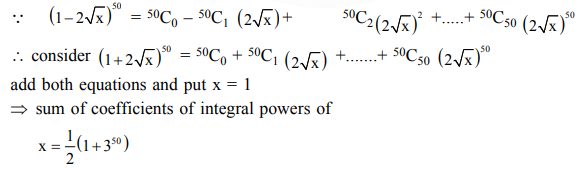# The sum of coefficients of integral powers of x in the binomial expansion of`
Question:

The sum of coefficients of integral powers of $x$ in the binomial expansion of $(1-2 \sqrt{x})^{50}$ is :

1. $\frac{1}{2}\left(3^{50}-1\right)$

2.  $\frac{1}{2}\left(2^{50}+1\right)$

3. $\frac{1}{2}\left(3^{50}+1\right)$

4. $\frac{1}{2}\left(3^{50}\right)$

Correct Option: , 3

Solution: